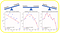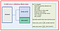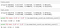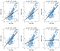# 7. Variance ErrorIllustration of bias error (underfit) and variance error (overfit). Image source: Simplicity vs. Complexity in Machine Learning — Finding the Right Balance, Benjamin O. Tayo

# 8. Random Errork-fold cross-validation pseudocode. Photo by Benjamin O. TayoSample R2 outputs from k-fold cross-validation calculation. Source: Hands-on k-fold Cross-validation for Machine Learning Model Evaluation — Cruise Ship Dataset, Benjamin O. Tayo

# 9. Error from Hyperparameter TuningRegression analysis using different values of the learning rate parameter. Source: Bad and Good Regression Analysis, Published in Towards AI, February 2019, by Benjamin O. Tayo.
`Perceptron(n_iter=40, eta0=0.1, random_state=0)train_test_split( X, y, test_size=0.4, random_state=0)LogisticRegression(C=1000.0, random_state=0)KNeighborsClassifier(n_neighbors=5, p=2, metric='minkowski')SVC(kernel='linear', C=1.0, random_state=0)DecisionTreeClassifier(criterion='entropy',                        max_depth=3, random_state=0)Lasso(alpha = 0.1)PCA(n_components = 4)`

# 10. Model Selection Error

`LogisticRegression()KNeighborsClassifier()SVC()DecisionTreeClassifier()RandomForestClassifier()GaussianNB()`

# Additional Data Science/Machine Learning Resources

Written by

Written by

## Benjamin Obi Tayo Ph.D.

#### Physicist, Data Science Educator, Writer. Interests: Data Science, Machine Learning, AI, Python & R, Predictive Analytics, Materials Sciences, Biophysics